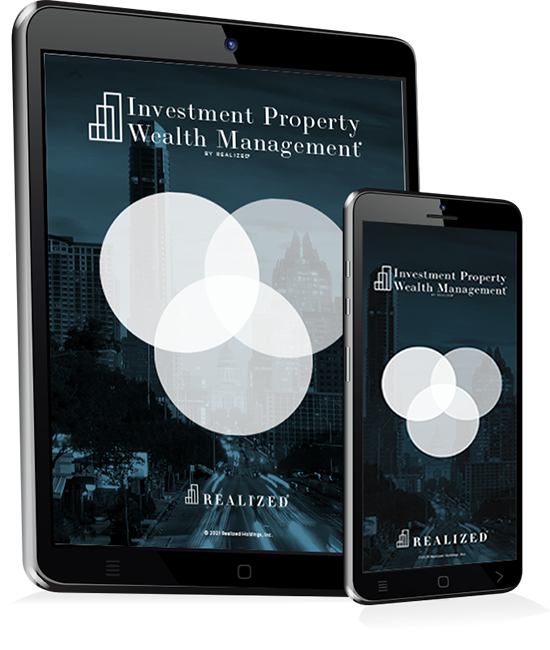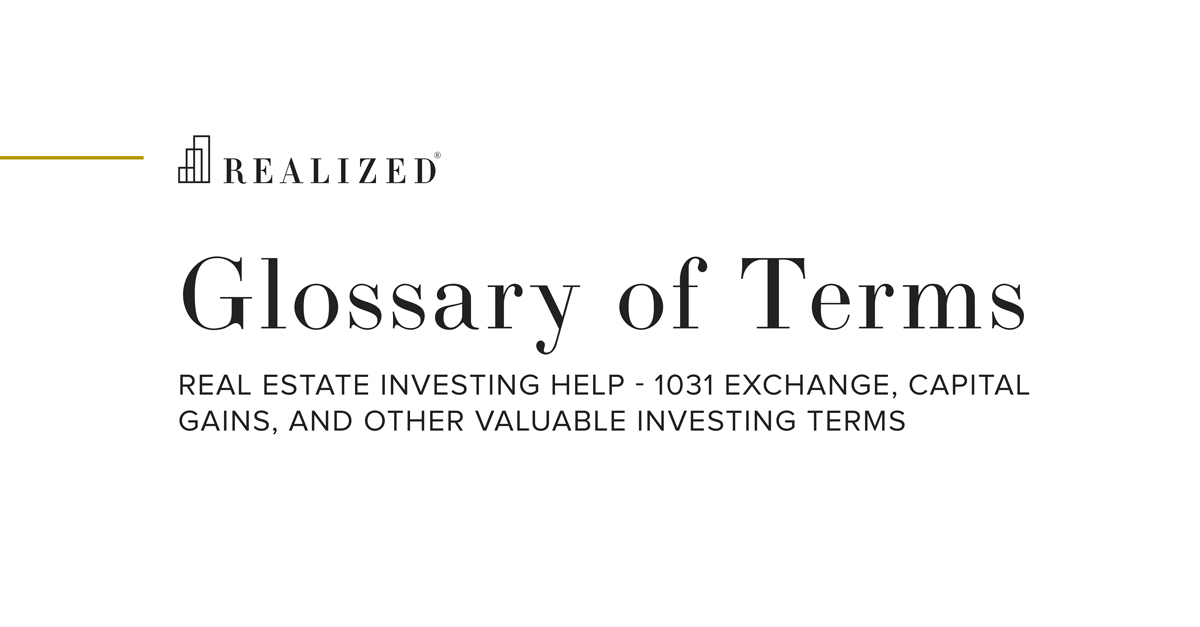Gross Rent Multiplier (GRM) 2015-09-16 08:00:00

An investment property valuation method which is the ratio of a property’s price to its gross revenue. A gross rent multiplier represents the time a property would take to pay for itself in gross income received. To calculate the gross rent multiplier (GRM) use the formula of sales price divided by gross monthly (or annual) rent. For example, if a property’s sale price is \$1,000,000 and the annual gross rent is \$80,000, then the gross rent multiplier is 12.5, meaning it would take 12.5 years of gross rents to equal the property’s value. A lower gross rent multiplier, indicates less time required to collect gross rents equal to purchase price, but may also indicate a higher risk profile. The gross rent multiplier technique measures investment real estate value based on gross revenue, which contrast with another valuation metric, cap rates, which determine value based on net income. The GRM method is often used in lieu of a cap rate when operating expenses are unknown or where an investor believes they can operate the property at a different level of expenses than the seller, and thus net income and cap rate methods may not be indicative of the property’s true value.